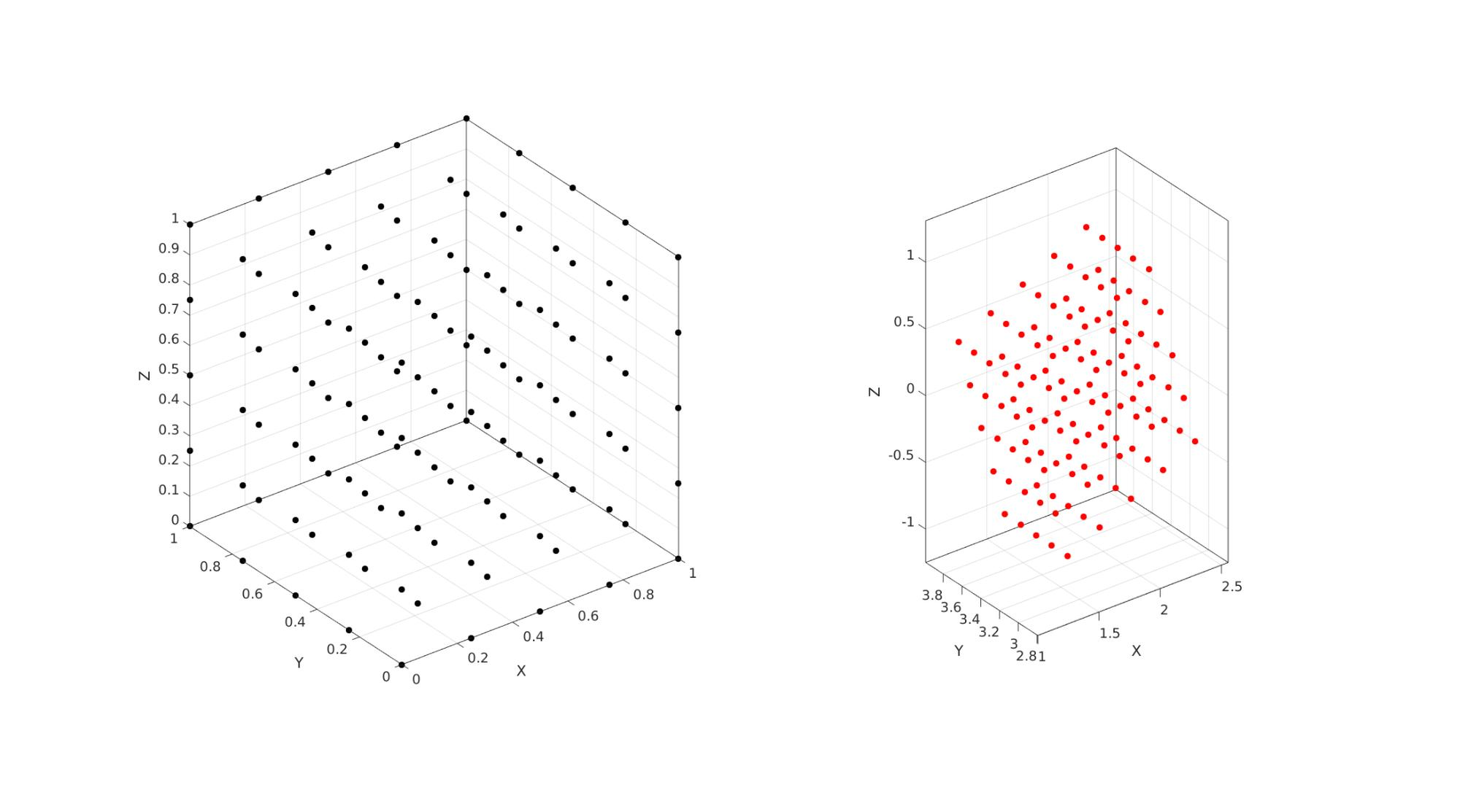# disp2strain

Below is a demonstration of the features of the disp2strain function

## Contents

```clear; close all; clc;
```

## Syntax

[D_out]=disp2strain(Ux, Uy, Uz, v, strain_type);

## Description

Computes deformation metrics (including strains) for the meshgrid formatted displacement arrays Ux, Uy, and Uz, based on the point spacing v and strain_type requested.

## Examples

```n=5; %Number of points defined in range
r=linspace(0,1,n); %The range used here
v=mean(diff(r))*ones(1,3); %The "voxel" or step size
[X1,Y1,Z1]=meshgrid(r); %Meshgrid formatted coordinate set

testCase=1;
switch testCase
case 1 % This uses a specified left stretch tensor
% Stretches (non-ordered)
l1=1.1; l2=0.7; l3=1.5;

%Build left stretch tensor
V=[l1 0  0;...
0  l2 0;...
0  0  l3];

%Define rotation
R=euler2DCM([0.25*pi 0.25*pi 0]);

F=V*R;

U=R'*F; %Right stretch tensor

%Define translation
t=[1 pi -0.5];
case 2 % This uses a specified right stretch tensor
%Stretches (non-ordered)
l1=1.1; l2=0.7; l3=1.5;

%Build right stretch tensor
U=[l1 0  0;...
0  l2 0;...
0  0  l3];

%Define rotation
R=euler2DCM([0.25*pi 0.25*pi 0]);

F=R*U;

V=F*R';% Left stretch tensor

%Define translation
t=[1 pi -0.5];
end

%Define initial coordinate vector
V1=[X1(:) Y1(:) Z1(:)];

%Create deformed coordinate vector
V2=(F*V1')'; %Rotate/stretch using deformation gradient tensor
V2=V2+t(ones(size(V2,1),1),:); %Apply translation

%
X2=reshape(V2(:,1),size(X1));
Y2=reshape(V2(:,2),size(X1));
Z2=reshape(V2(:,3),size(X1));

%Compute displacements
Uxyz=V2-V1;

Ux=X2-X1; Uy=Y2-Y1; Uz=Z2-Z1;
```

Compute deformation data from displacements

```% 1 = Biot (linear) strain tensor
% 2 = Hencky (logarithmic/natural) strain tensor
% 3 = Green-Lagrange strain tensor
strain_type=1;
[outputStructure]=disp2strain(Ux, Uy, Uz, v, strain_type);

%Check output against input
F
F_check=outputStructure.F{1}

R
R_check=outputStructure.R{1}

U
U_check=outputStructure.U{1}

V
V_check=outputStructure.V{1}
```
```F =

0.7778         0    0.7778
0.3500    0.4950   -0.3500
-0.7500    1.0607    0.7500

F_check =

0.7778         0    0.7778
0.3500    0.4950   -0.3500
-0.7500    1.0607    0.7500

R =

0.7071         0    0.7071
0.5000    0.7071   -0.5000
-0.5000    0.7071    0.5000

R_check =

0.7071    0.0000    0.7071
0.5000    0.7071   -0.5000
-0.5000    0.7071    0.5000

U =

1.1000   -0.2828         0
-0.2828    1.1000    0.2828
0    0.2828    1.1000

U_check =

1.1000   -0.2828    0.0000
-0.2828    1.1000    0.2828
0.0000    0.2828    1.1000

V =

1.1000         0         0
0    0.7000         0
0         0    1.5000

V_check =

1.1000    0.0000    0.0000
0.0000    0.7000    0.0000
0.0000    0.0000    1.5000

```
```cFigure;
subplot(1,2,1); hold on;
plot3(X1(:),Y1(:),Z1(:),'k.','MarkerSize',25);
axisGeom;

subplot(1,2,2); hold on;
plot3(X2(:),Y2(:),Z2(:),'r.','MarkerSize',25);
axisGeom;

gdrawnow;
```GIBBON www.gibboncode.org

Kevin Mattheus Moerman, [email protected]

GIBBON footer text

GIBBON: The Geometry and Image-based Bioengineering add-On. A toolbox for image segmentation, image-based modeling, meshing, and finite element analysis.

Copyright (C) 2006-2023 Kevin Mattheus Moerman and the GIBBON contributors

This program is free software: you can redistribute it and/or modify it under the terms of the GNU General Public License as published by the Free Software Foundation, either version 3 of the License, or (at your option) any later version.

This program is distributed in the hope that it will be useful, but WITHOUT ANY WARRANTY; without even the implied warranty of MERCHANTABILITY or FITNESS FOR A PARTICULAR PURPOSE. See the GNU General Public License for more details.

You should have received a copy of the GNU General Public License along with this program. If not, see http://www.gnu.org/licenses/.